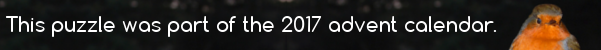mscroggs.co.uk
mscroggs.co.uksubscribe

# Puzzles

## 4 DecemberToday's number is the number of 0s that 611! (611×610×...×2×1) ends in.

## Square and cube endings

Source: UKMT 2011 Senior Kangaroo
How many positive two-digit numbers are there whose square and cube both end in the same digit?

## Digitless factor

Ted thinks of a three-digit number. He removes one of its digits to make a two-digit number.
Ted notices that his three-digit number is exactly 37 times his two-digit number. What was Ted's three-digit number?

## Backwards fours

If A, B, C, D and E are all unique digits, what values would work with the following equation?
$$ABCCDE\times 4 = EDCCBA$$

## 11 DecemberTwo more than today's number is the reverse of two times today's number.

## 4 DecemberPick a three digit number whose digits are all different.
Sort the digits into ascending and descending order to form two new numbers. Find the difference between these numbers.
Repeat this process until the number stops changing. The final result is today's number.

## 1 DecemberToday's number is the smallest three digit number such that the sum of its digits is equal to the product of its digits.

## XYZ

Which digits $$X$$, $$Y$$ and $$Z$$ fill this sum?
$$\begin{array}{cccc} &X&Z&Y\\ +&X&Y&Z\\ \hline &Y&Z&X \end{array}$$

## Archive

Show me a random puzzle
▼ show ▼